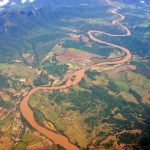Want to share your content on R-bloggers? click here if you have a blog, or here if you don't.R FOR HYDROLOGISTS

CORRELATION AND INFORMATION THEORY MEASUREMENTS – PART 3

Before we begin, if you don’t have the data, first get it from the first tutorial here. You will also need to Install and load the `ggplot2` and `reshape2` packages.
``` if(!require(ggplot2)){install.packages(ggplot2, dep=T)} if(!require(reshape2)){install.packages(reshape2, dep=T)} ```
Answers to these exercises are available here.

The mutual information quantifies the “amount of information” between the two variables in bits. To transform it into a metric, there has been several variants proposed of the MI; one of those is a normalization that assumes MI as an analog of co-variance and calculated it as a Pearson correlation co-efficient `NMI=MI/(Hx+Hy)^(1/2)`.

Exercise 1
Please write a function to calculate the normalized mutual information with two input parameters `x,y` as vectors and NMI as the return value. Hint: Reuse the code of the last tutorial .

Exercise 2
Similar to before, we will estimate the linear auto-correlation function. Also, it is possible to estimate a nonlinear auto-correlation function using the NMI as a correlation co-efficient of the lags of the time series. Please load the function `createLags(x, numberOfLags, VarName)` and create the embedded space for the first 400 lags of the `LEVEL` and the `RAIN`.

Exercise 3
To calculate the nonlinear auto-correlation function (NACF), you can estimate the NMI for the first column of `lags_level` compared with all the other lags. Do it also for the `lags_rain`.

Exercise 4
To calculate the nonlinear cross correlation function (NCCF), you can estimate the NMI for the first column of `lags_level` compared with all the lags of `lags_rain`. Do it also for the `lags_rain` compared with all the lags of ` lags_level `.

Learn more about Model Evaluation in the online course Regression Machine Learning with R. In this course, you will learn how to:

• Avoid model over-fitting using cross-validation for optimal parameter selection
• Use correlation to avoid multi-col linearity problems in your model
• And much more

Exercise 5
Another very useful tool of measurement is the the Kullback–Leibler divergence or relative entropy. It measures how one’s probability distribution `q` diverges from a second expected probability distribution `p` . It is possible to estimate it using the formula: cross entropy of `q` respect to `p` minus the entropy of `p`.
To estimate the probability distribution `p` and `q`, this time we will change our approach and we will use a `geom_histogram`. Please create a histogram of 10 bins from the level and a histogram from the first lag, then assign it to `p` and `q`.
Hint: 1) Remember to always use the interval from p for the histograms. 2) After grabbing the first layer of data from the plot with ` layer_data `, you can get use from the column `\$count `.

Exercise 6
Now, please calculate the entropy of `p`.

Exercise 7
Now calculate the cross entropy ` Hp_q` with the formula `-sum(p*log2(q))`. Hint: Remember to avoid negative values of q.

Exercise 8
Finally, please calculate and print the Kullback–Leibler divergence.